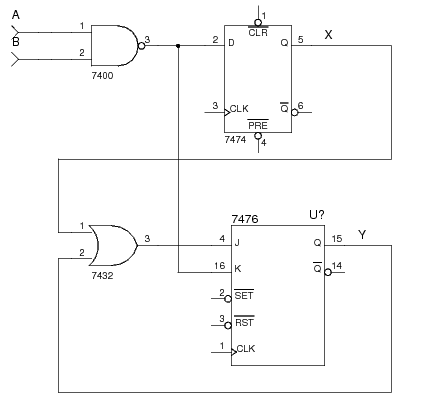# Flip-Flop Input Equation

The combinational logic circuit inputs to a flip-flop are described by a flip-flop input Boolean equation.

## Example

Consider the following sequential logic circuit containing a JK flip-flop and a D flip-flop (not all connections are shown to keep the schematic simple):The D flip-flop has output `X`. Its input has no name, so let's call its input `DX`. The JK flip-flop has output `Y`. Its inputs also have no names so let's call them `JY`, and `KY`. We can then give formulas for the combinational logic inputs of each flip-flop input as follows:

• `DX = A nand B`
• `JY = X or Y`
• `KY = A nand B`

## References

Mano, M. Morris, and Kime, Charles R. Logic and Computer Design Fundamentals. 2nd Edition. Prentice Hall, 2000.